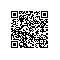# beamer 中使用 tikz 画图和动画

[cce lang=’latex’]
\usepackage{tikz}

\tikzstyle{block} = [rectangle, draw, fill=blue!20, text width=4em, text centered, rounded corners]
\tikzstyle{hugeBlock} = [rectangle, draw, fill=blue!20,
text width=5em, text centered, rounded corners, minimum height=4em]
\tikzstyle{line} = [draw, -latex’]
[/cce]

[cce lang=’latex’]
\begin{frame}{集中式工作流}

\begin{tikzpicture}[node distance = 2cm, auto]
\node [block] (center) {共享仓库};
\node[block, below of=center, node distance = 3cm](centerDevelop){开发者2};
\node[block, left of=centerDevelop, node distance = 3cm](leftDevelop){开发者1};
\node[block, right of=centerDevelop,node distance = 3cm](rightDevelop){开发者3};
\path[line,<->](center) — (leftDevelop);
\path[line,<->](center) — (centerDevelop);
\path[line,<->](center) — node{push/pull} (rightDevelop);
\end{tikzpicture}
\end{frame}
[/cce][cce lang=’latex’]
\begin{frame}\frametitle{集成管理员工作流}\framesubtitle{参与者流程}
\begin{tikzpicture}[node distance = 3cm, auto]
\path[use as bounding box] (-1,0) rectangle (10,-2);
\path[line]<1-> node[block](fock){fock工程};
\path[line]<2-> node[block, right of=fock, node distance=3cm](clone){克隆到本地}
(fock) — (clone);
\path[line]<3-> node[block, right of=clone, node distance=3cm](edit){修改}
(clone) — (edit);
\path[line]<4-> node[block, below of=edit, node distance=3cm](commit){提交}
(edit) — (commit);
\path[line]<5-> node[block, left of=commit, node distance=3cm](push){推送远程}
(commit) — (push);
\path[line]<6-> node[block, left of=push, node distance=3cm](mergeRequst){请求merge}
(push) — (mergeRequst);
\end{tikzpicture}
\end{frame}
[/cce]使用钉钉扫一扫加入圈子
+ 订阅